Multi-functionality with functClust

Repeated observations of a given performance or multi-functionality of a system

The inputs of fclust can include several observed performances generated by the same component assemblages. The different performances can correspond to a same system function or property observed at different times, in different places or under different environmental conditions. It is then repeated observations of a given system function or property. All observed performances are of same nature : they are equivalent and, generally, do not deserve to be differently weighted. This is for instance the biomass yearly harvested in 2004, 2005 and 2006 in Biodiversity II experiment done at Cedar Creek by David Tilman and his collaborators (“y2004”, “y2005” and “y2006” in CedarCreek.2004.2006.dat).

The different performances can also correspond to different functions or properties generated by a same, given collection of component assemblages belonging to a given system. The observed performances are of different nature, they are not equivalent, and each one deserves perhaps to be differently weighted. It is then about the understanding of multi-functionality of a system. This would be the case, for instance, for biomass of shoots and biomass of roots harvested for a given year in Biodiversity II experiment done at Cedar Creek.

Both the cases can be analysed by the functions fclust and plotted by the function fclust_plot. In the following, we term “experiment” the observation of a performance, whatever the performance, on a given collection of component assemblages belonging to a given system. If the inputs of fclust include several observed performances generated by the same component assemblages, the option xpr (for eXPeRiment) is activated in the code of functClust.

The option “weight” of fclust

The function fclust needs at least the name of dataset file and the number nbElt of components belonging to the interactive system in consideration (see vignette b.The simplest use of functClust ). The number nbXpr of observed performances is thus known: nbXpr = dim(dataset) - (1 + nbElt). If nbXpr is higher then 1, the option xpr is activated.

• weight : If there are several experiments, the option weight of function fclust must then be filled. weight is a vector of numerics, that specifies the weight to associate to each observed performance in the computations of both the quality criterion R2 (coefficient of determination of tree) and E (efficiency of tree). If the option weight is not filled, all observed performances are considered as equivalent (weight is equal to 1 for each experiment) (see Reference for more informations).
res <- fclust(dat, nbElt, weight = c(1, 1, 1), opt.mod = "byelt", opt.mean = "gmean")
fclust_plot(res, main = "BioDiv2", opt.tree = list("prd"))

res2 <- fclust(dat, nbElt, weight = c(2, 3, 1), opt.mod = "byelt", opt.mean = "gmean")
fclust_plot(res2, main = "BioDiv2", opt.tree = list("prd"))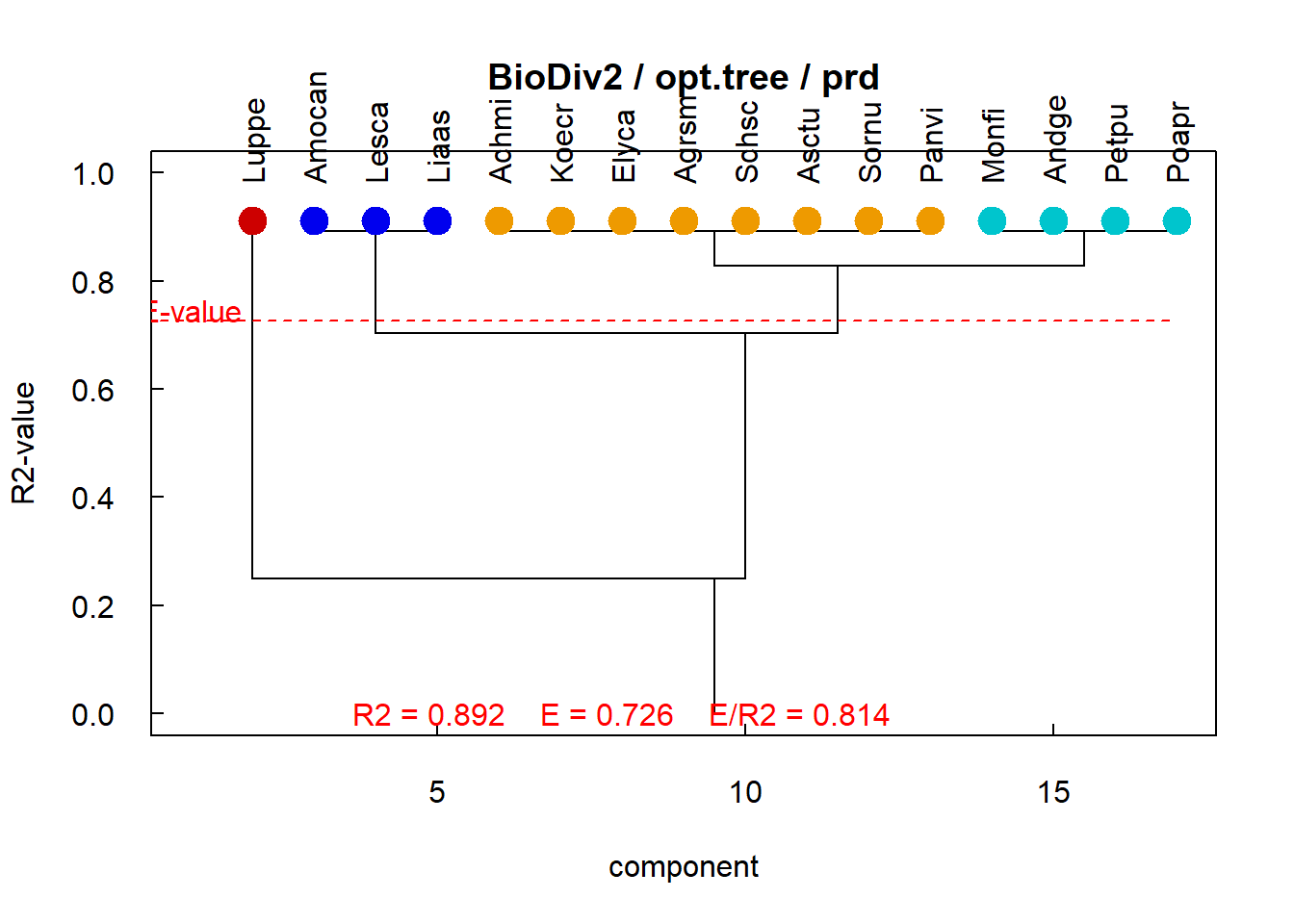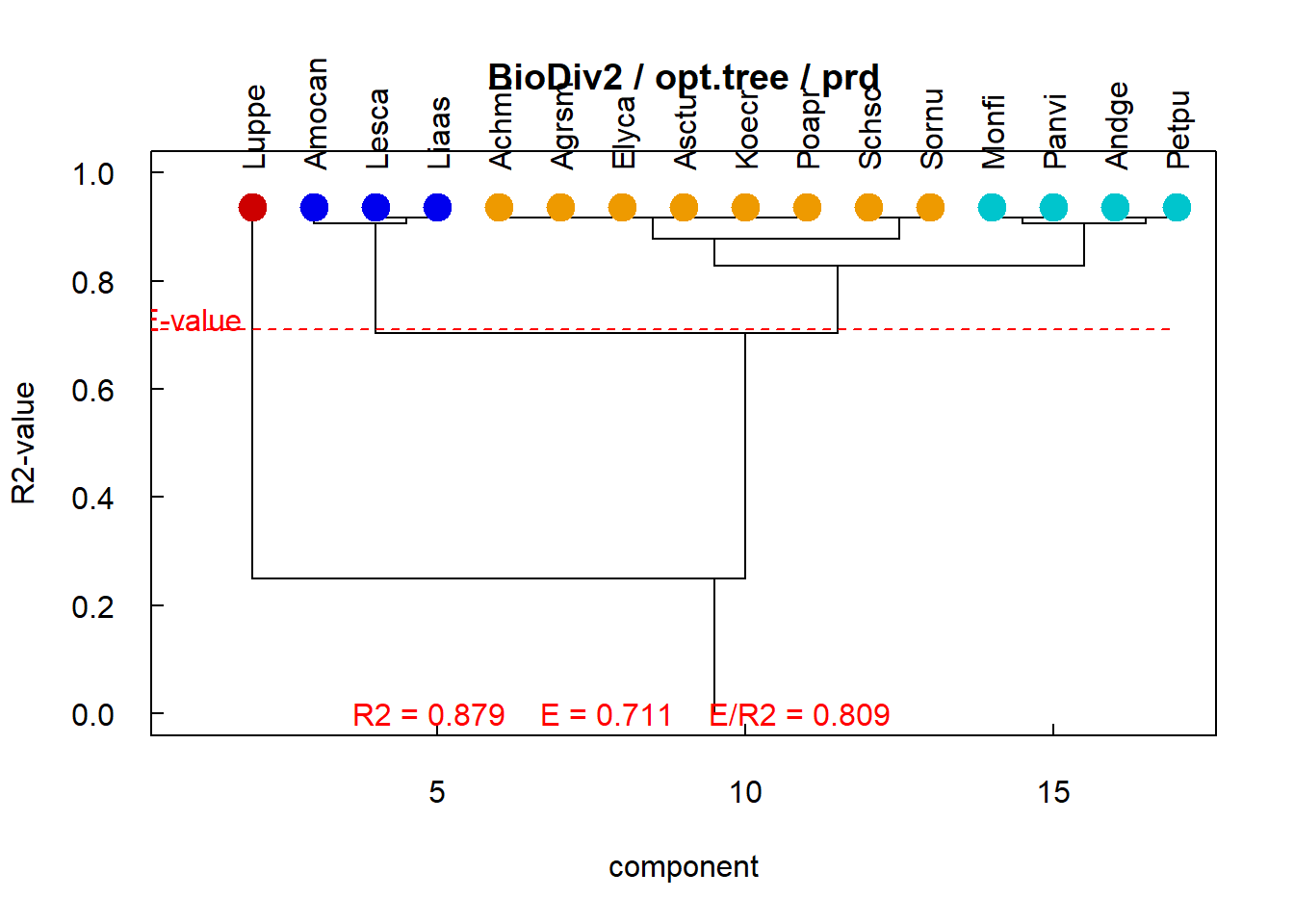Weight = c(1,1,1) on left, = c(2,3,1) on right tree

The options of fclust_plot

The function fclust returns un object that contains all necessary informations for plotting the results, especially the names of different experiments. If the number nbXpr of experiments is higher than 1, the option xpr is activated. Then, the user can require that the results of functional clustering are plotted as a whole (xpr=all) and experiment by experiment (xpr=names(xpr)). That only needs to add the option multi in the list of options. In the following, the changes are noted and illustrated for each option.

• opt.tree manages the plot of primary and secondary trees of component clustering. Any functional analysis generates only a primary and a secondary tree. The analysis of several performances does not change anything.

• opt.perf manages the plot of observed, modelled and predicted performances of assemblages Functional analysis generates only a primary and a secondary tree. The analysis of several performances does not change anything.

fclust_plot(res, main = "BioDiv2", opt.perf = list("multi"))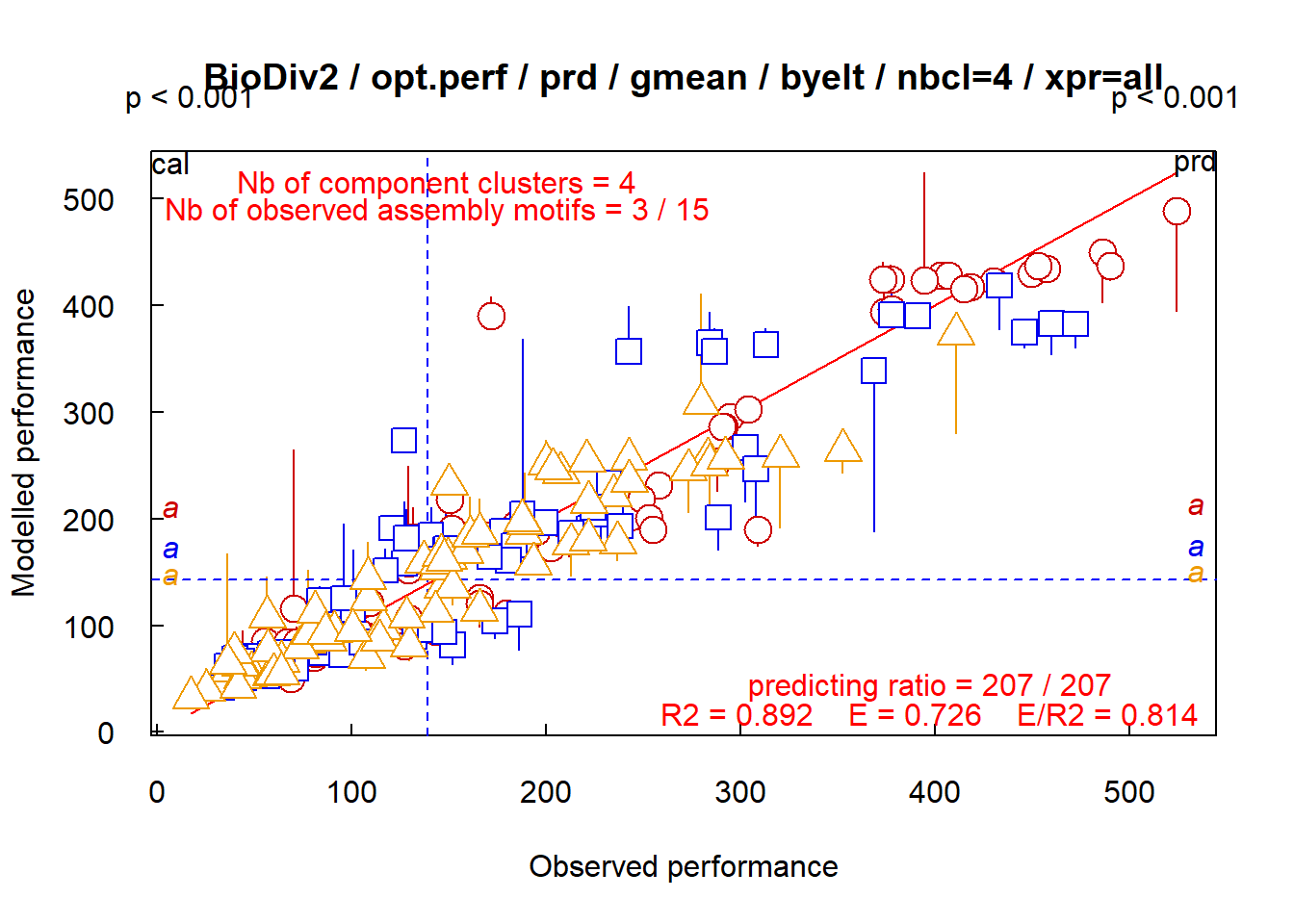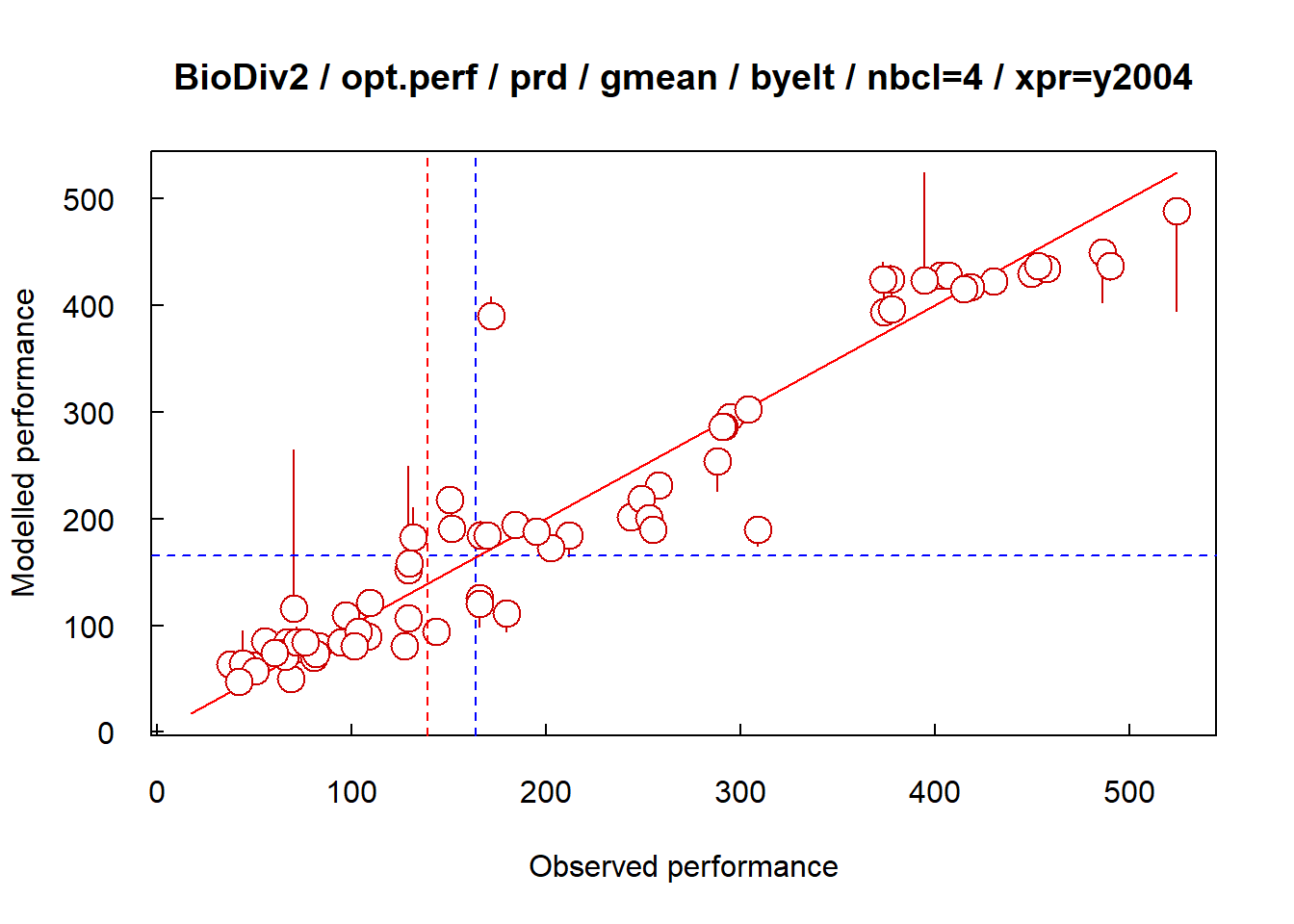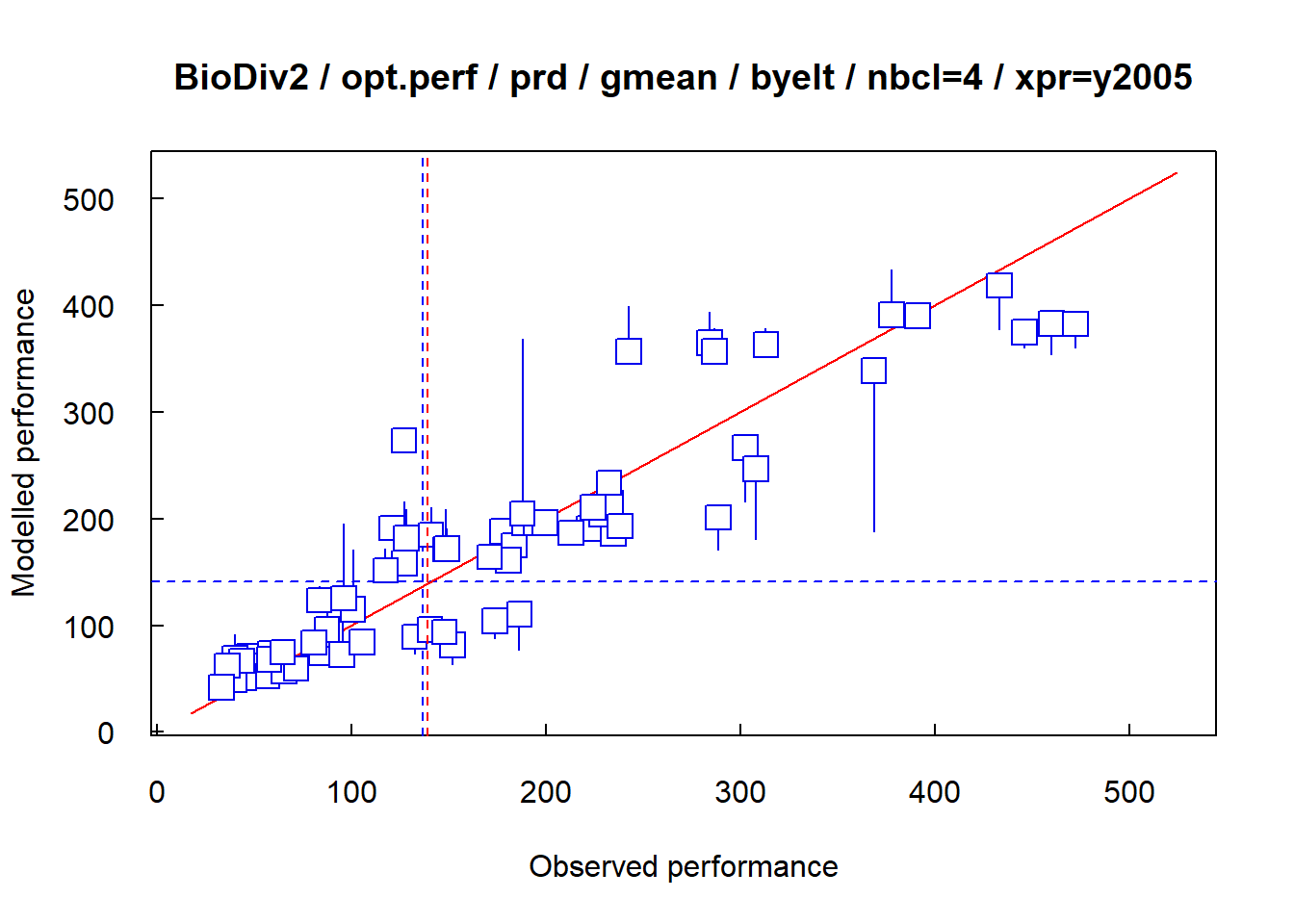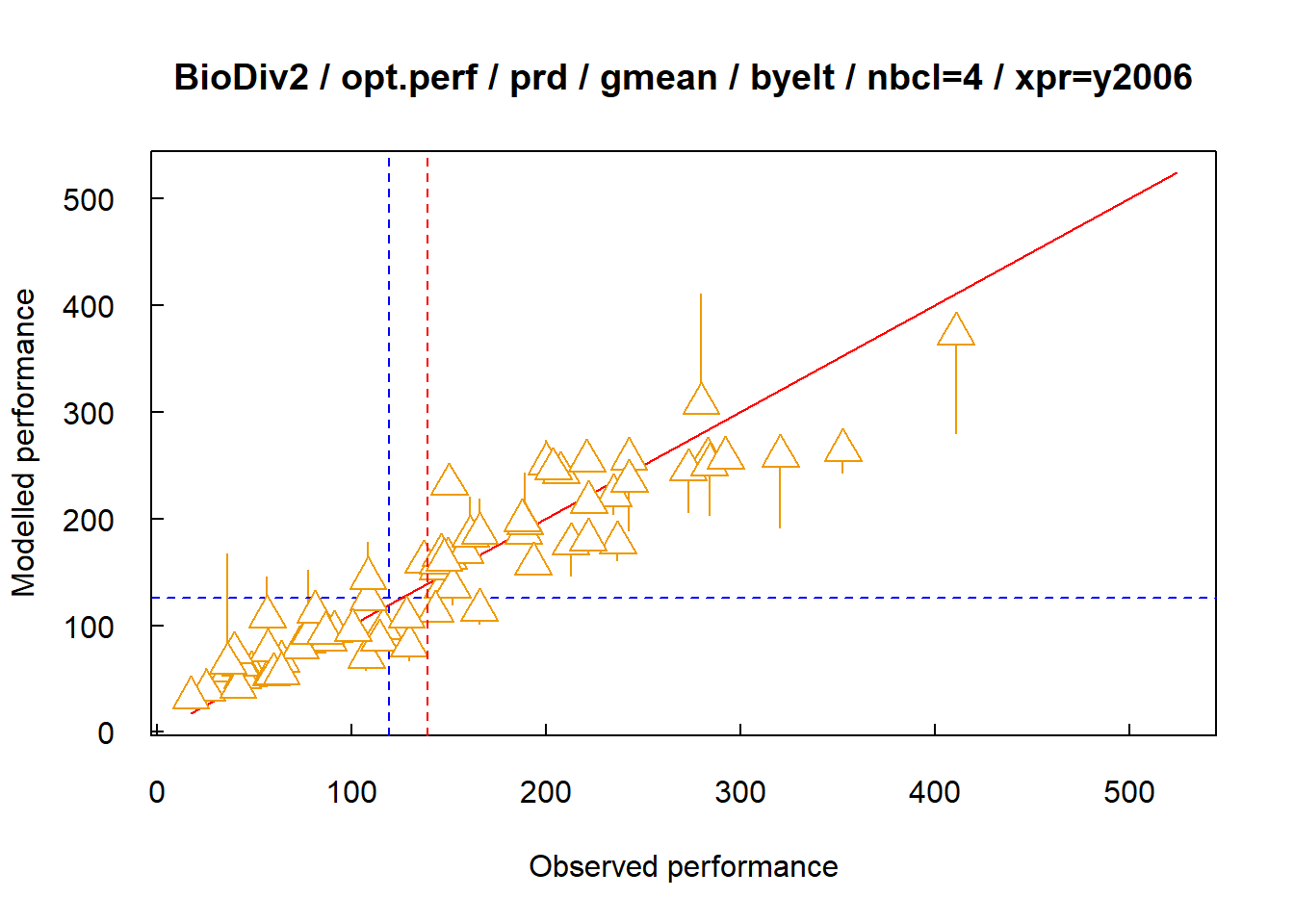Opt.perf = list(‘xpr’) plots the performances over time

• opt.ass plots different performances of some given, identified assemblages. The option is activated only when the option xpr is activated, that is when a same assemblage performance is observed over several experiments, at different times, places or environmental conditions, or when different assemblage performances are generated by given assemblages belonging to a system. Consequently, it is not necessary to specify multi in the list of options.
fclust_plot(res, main = "BioDiv2", opt.ass = list(who = c("20", "138")))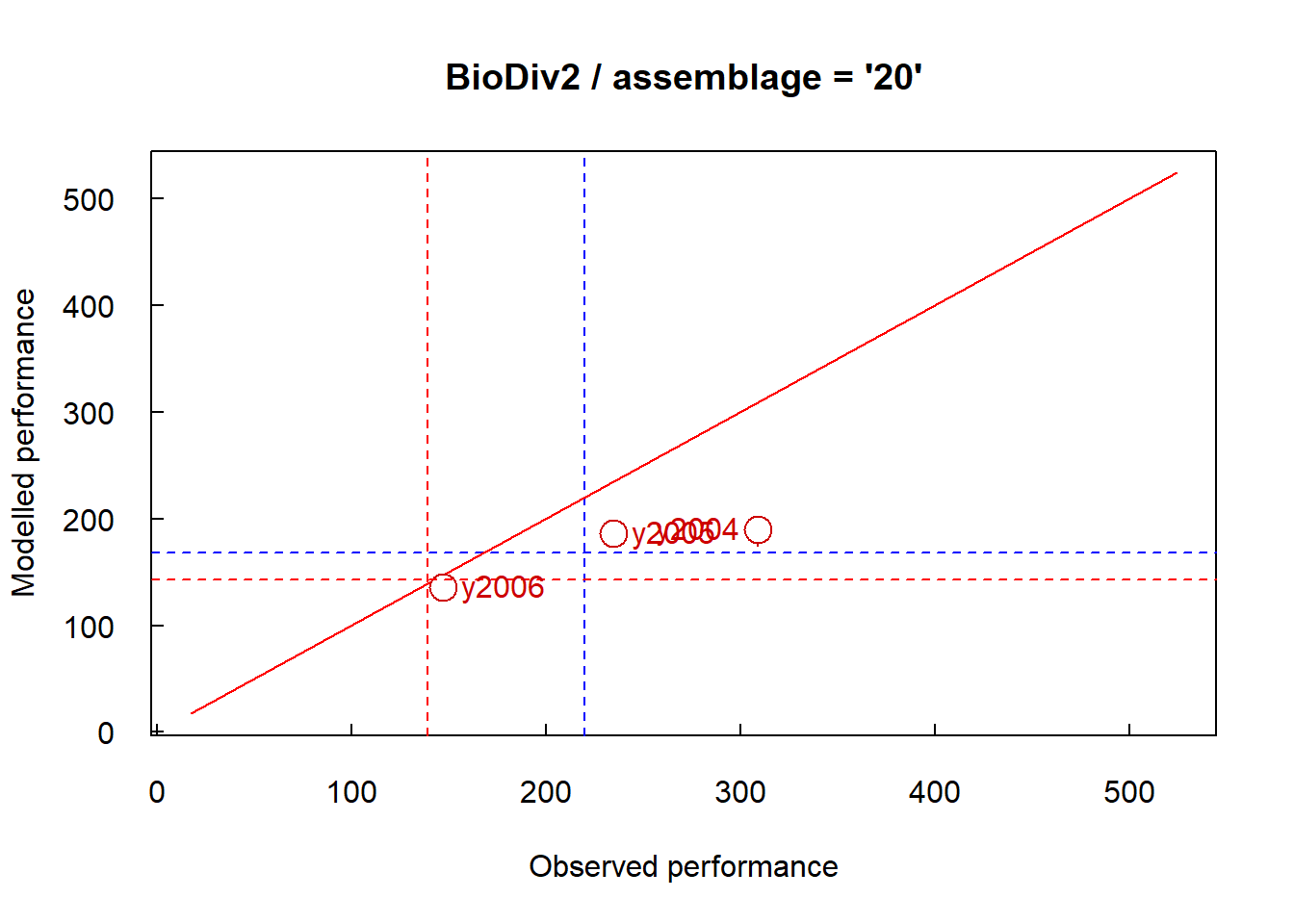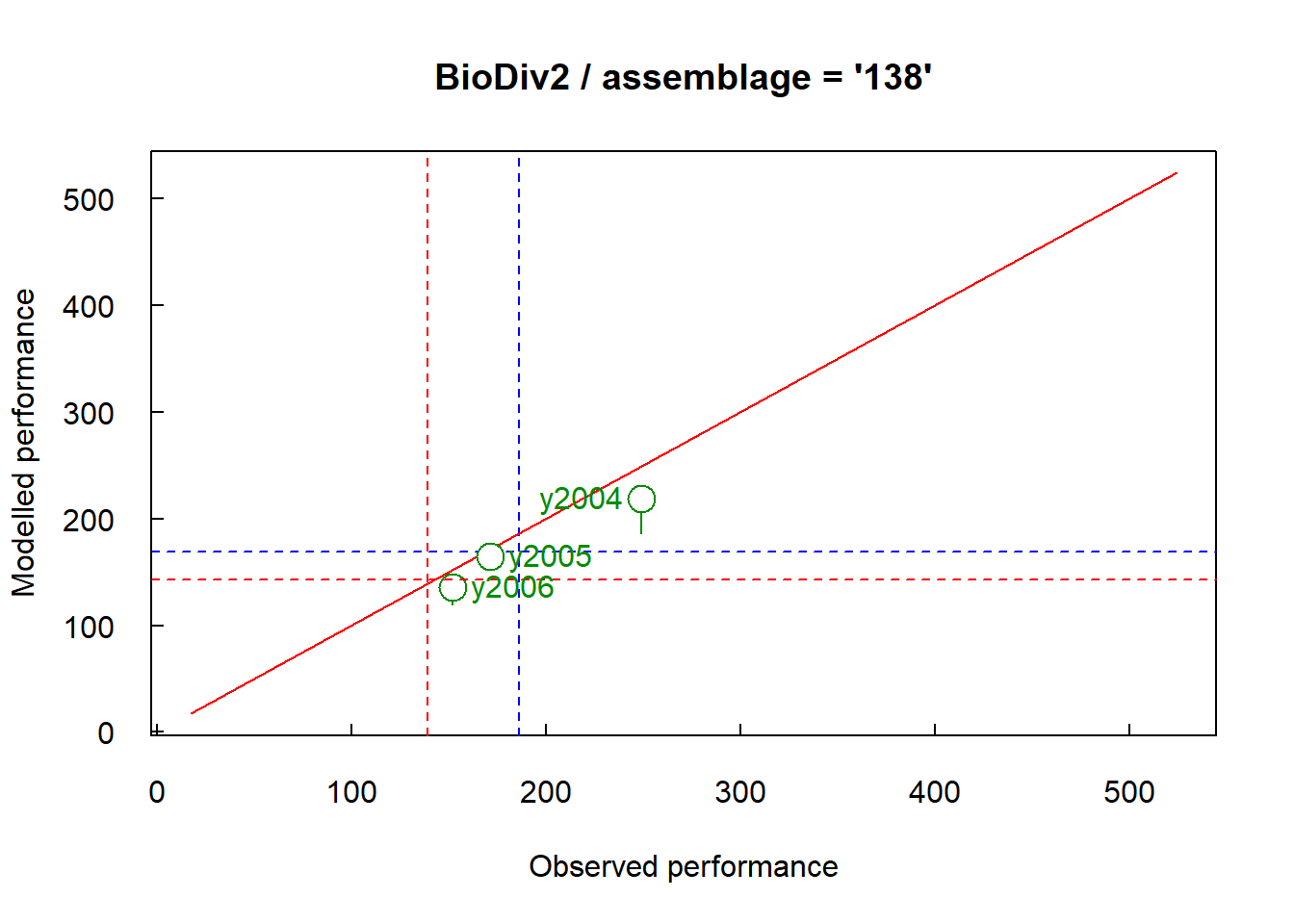Opt.ass = list(who = c(‘20’, ‘138’)) plots the performances over time of assemblages ‘20’ and ‘138’

• opt.motif manages the plot of mean performances of assembly motifs as boxplots. When the option xpr is activated, the options “obs”, “cal”, “prd” plot mean performances as a whole. To plot mean performances experiment by experiment, it is necessary to add the option multi in the list of options.
fclust_plot(res, main = "BioDiv2", opt.motif = list("prd", "hor", "multi", "aov", pvalue = 0.01))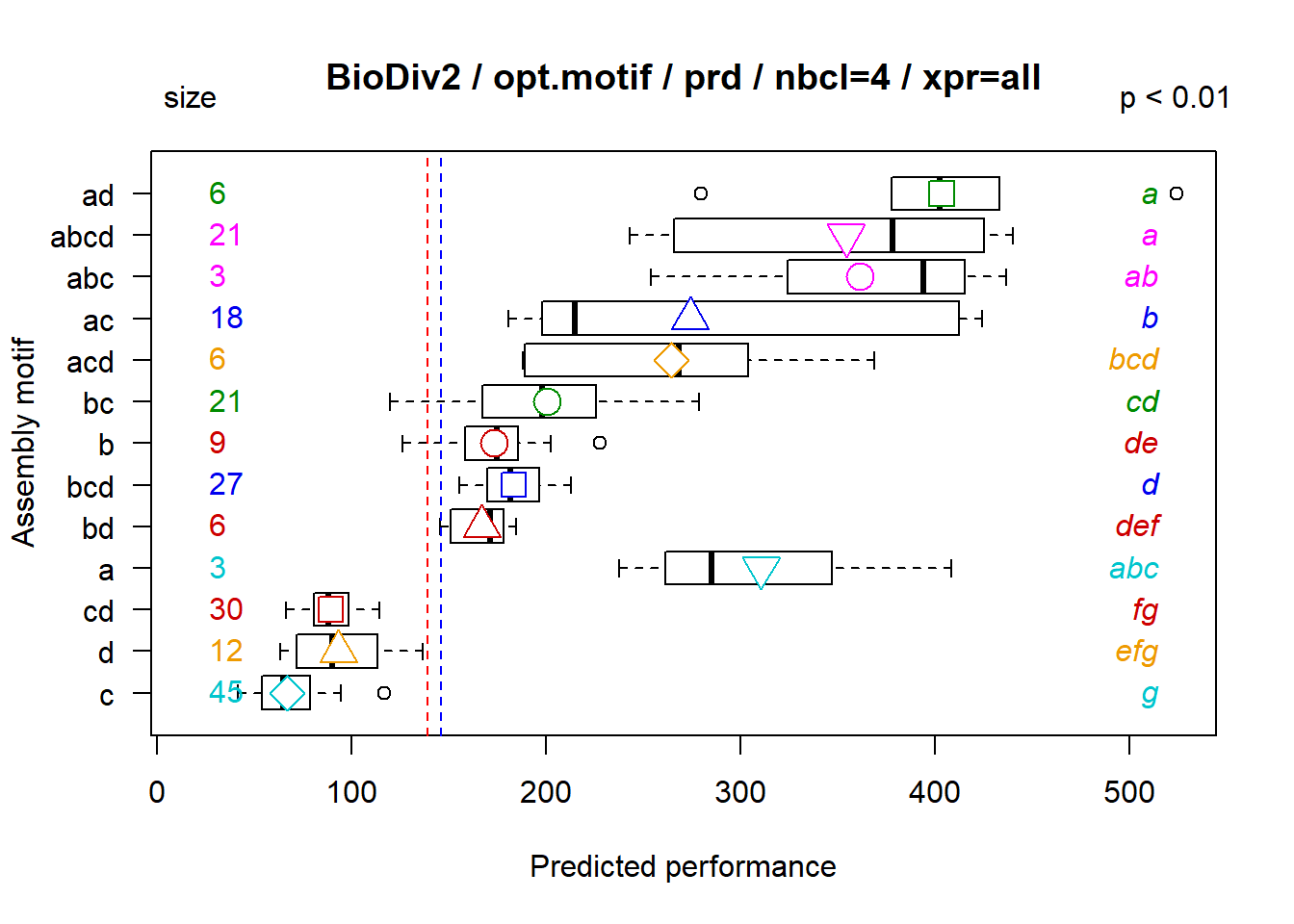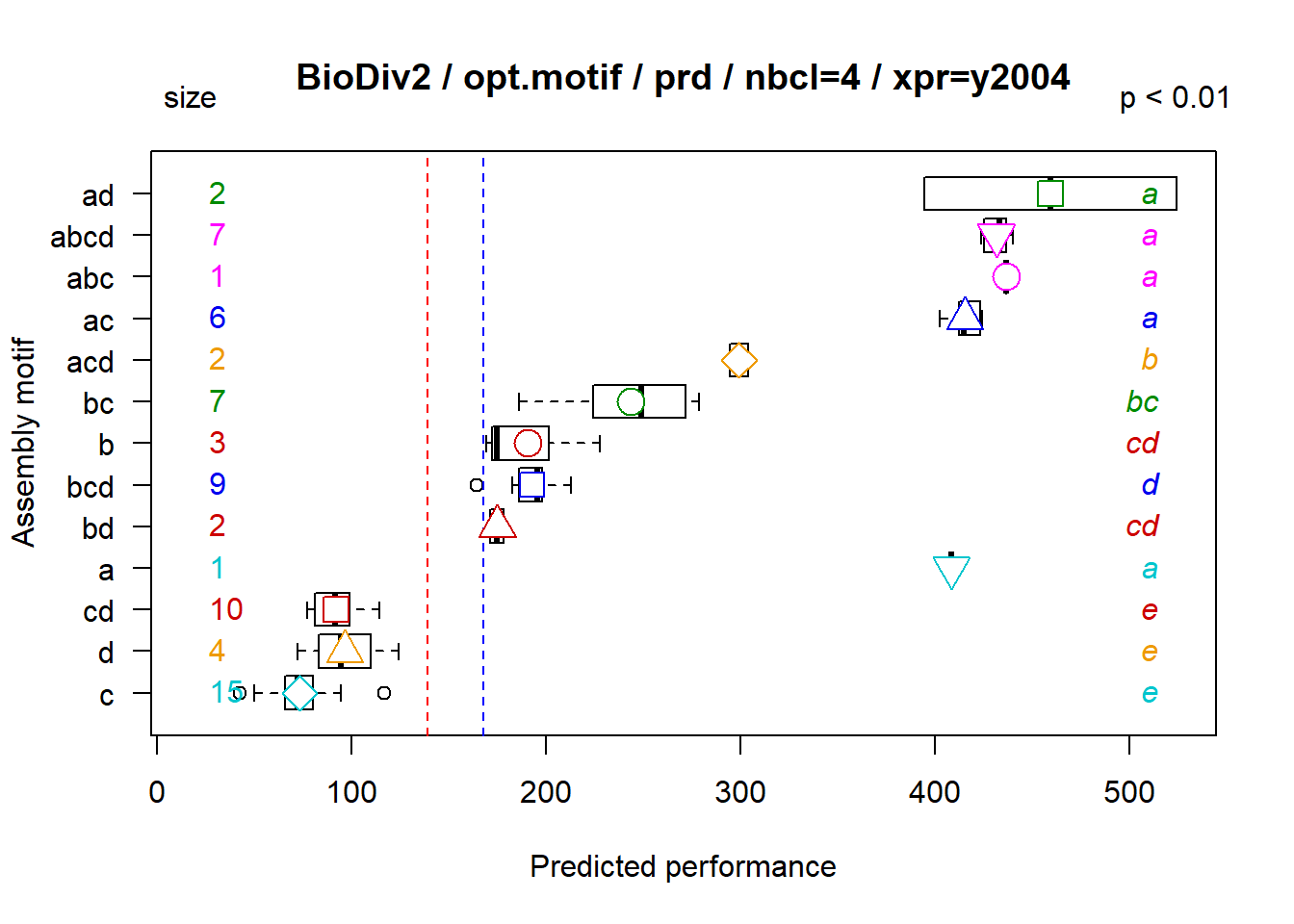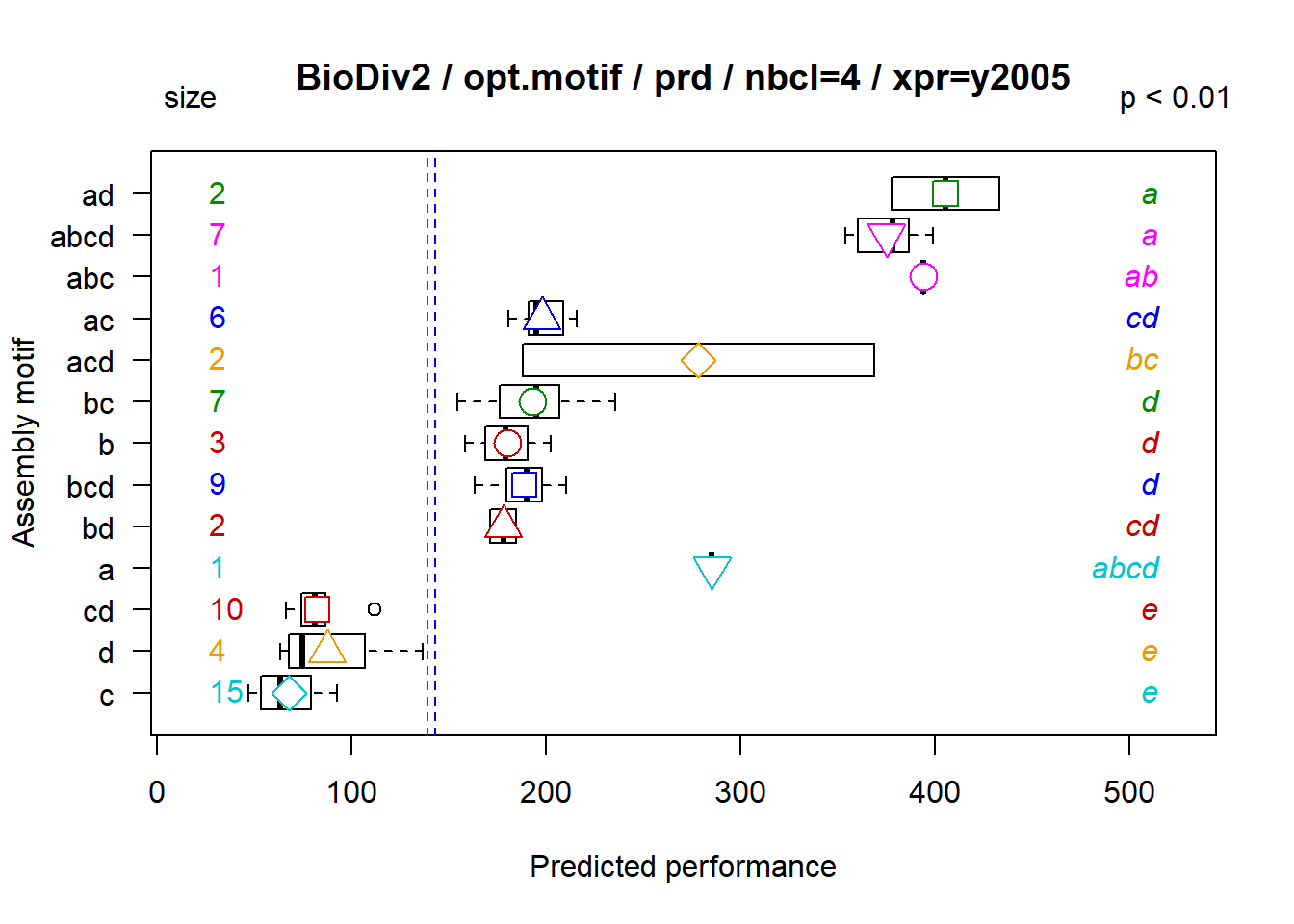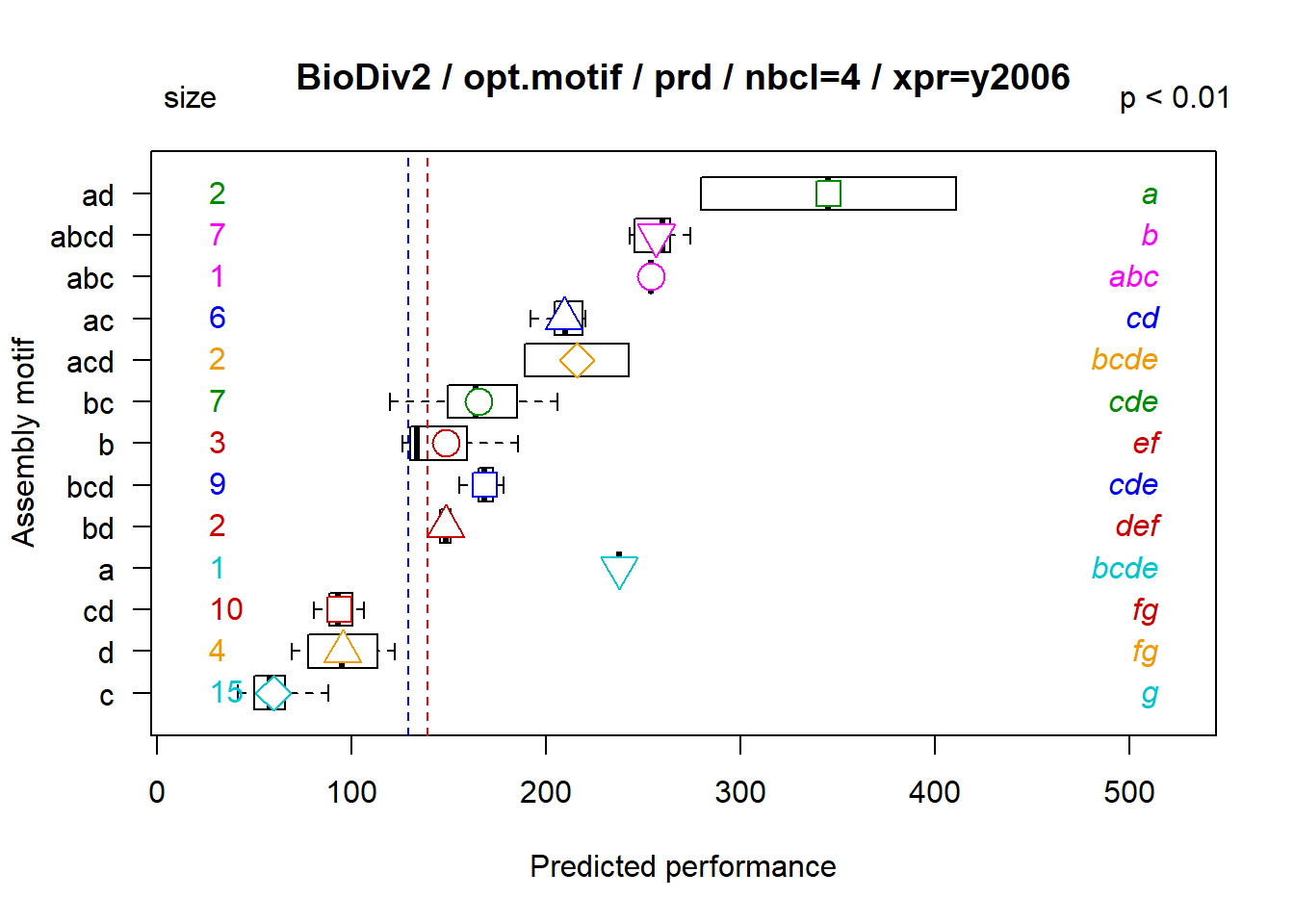Opt.motif = list(‘obs’, ‘seq’) plots performances over time sorted by assembly motif

• opt.comp manages the plot as boxplot of observed mean performances of assemblages that contain a given component. When the option xpr is activated, the options “tree”, “perf” plot mean performances as a whole. To plot mean performances experiment by experiment, it is necessary to add the option multi in the list of options.
fclust_plot(res, main = "BioDiv2", opt.comp = list("tree", "multi", "aov", pvalue = 0.01))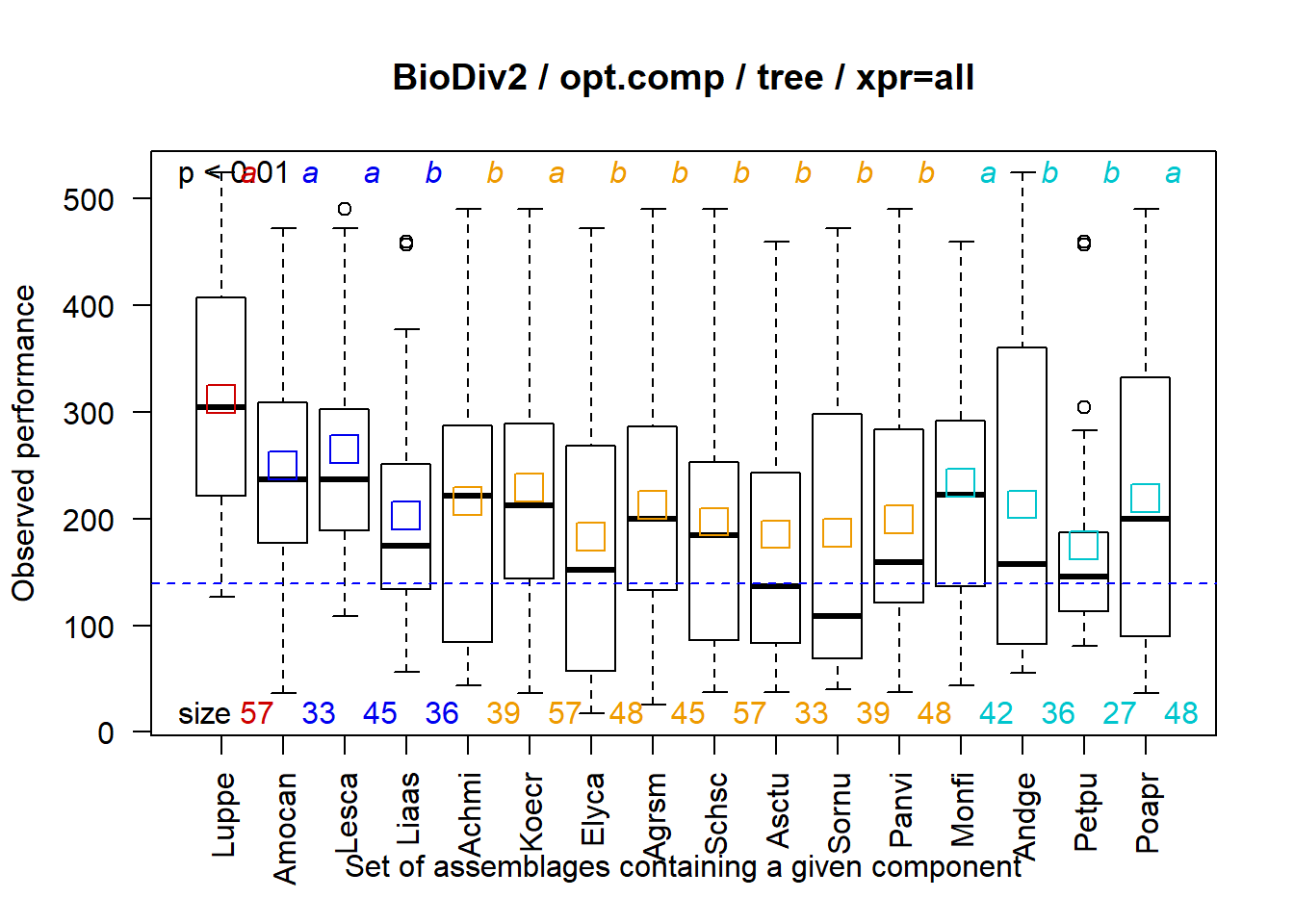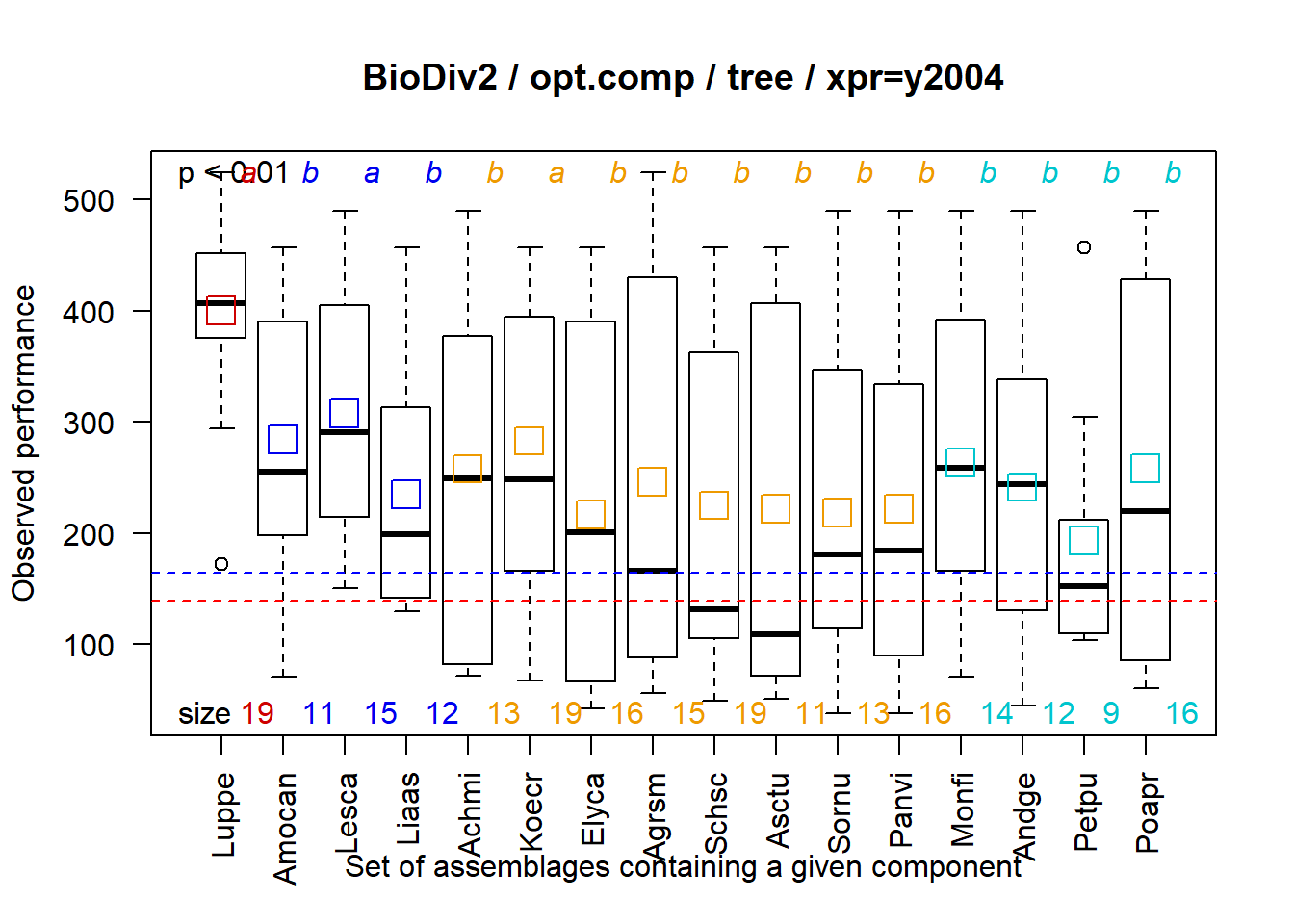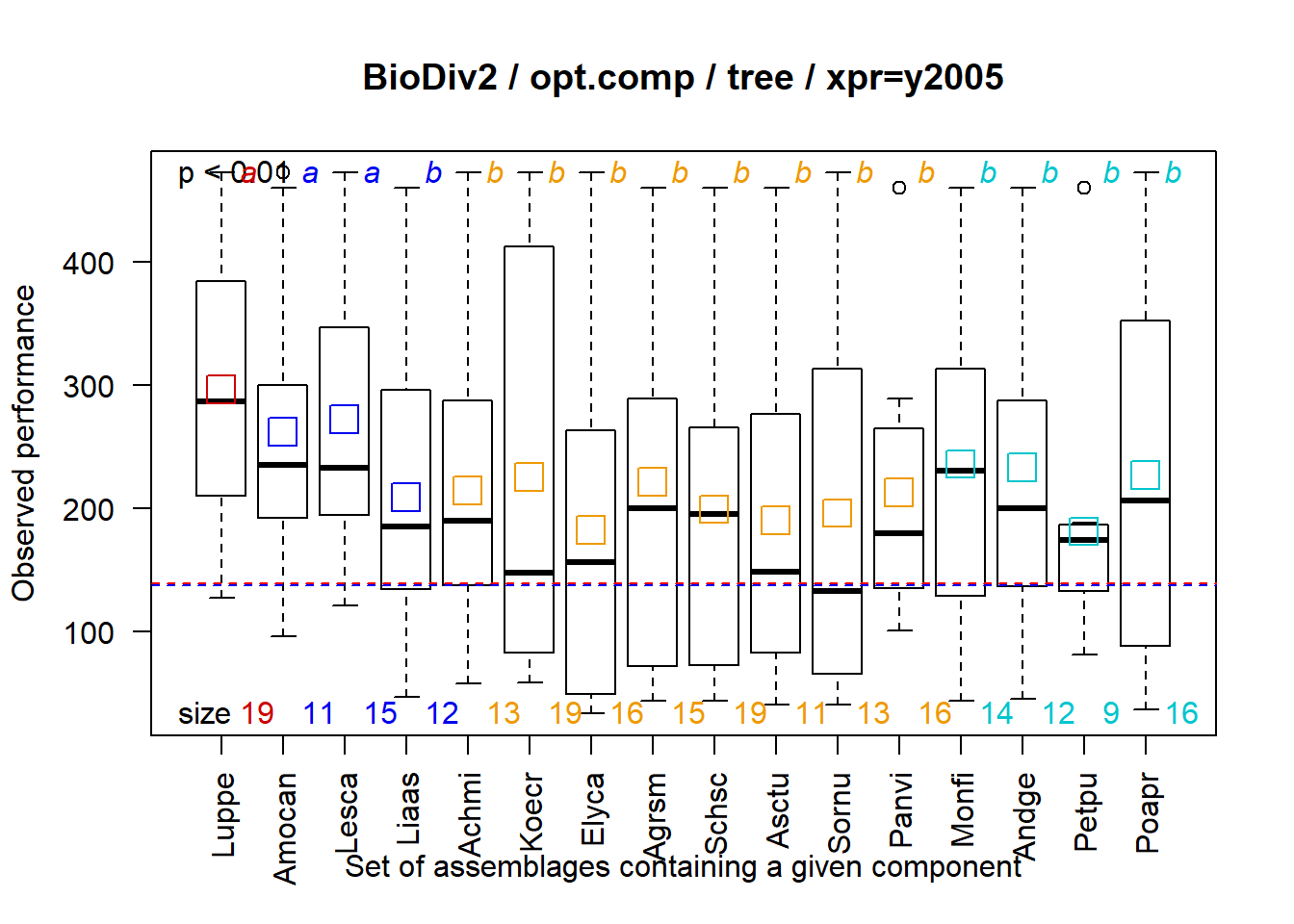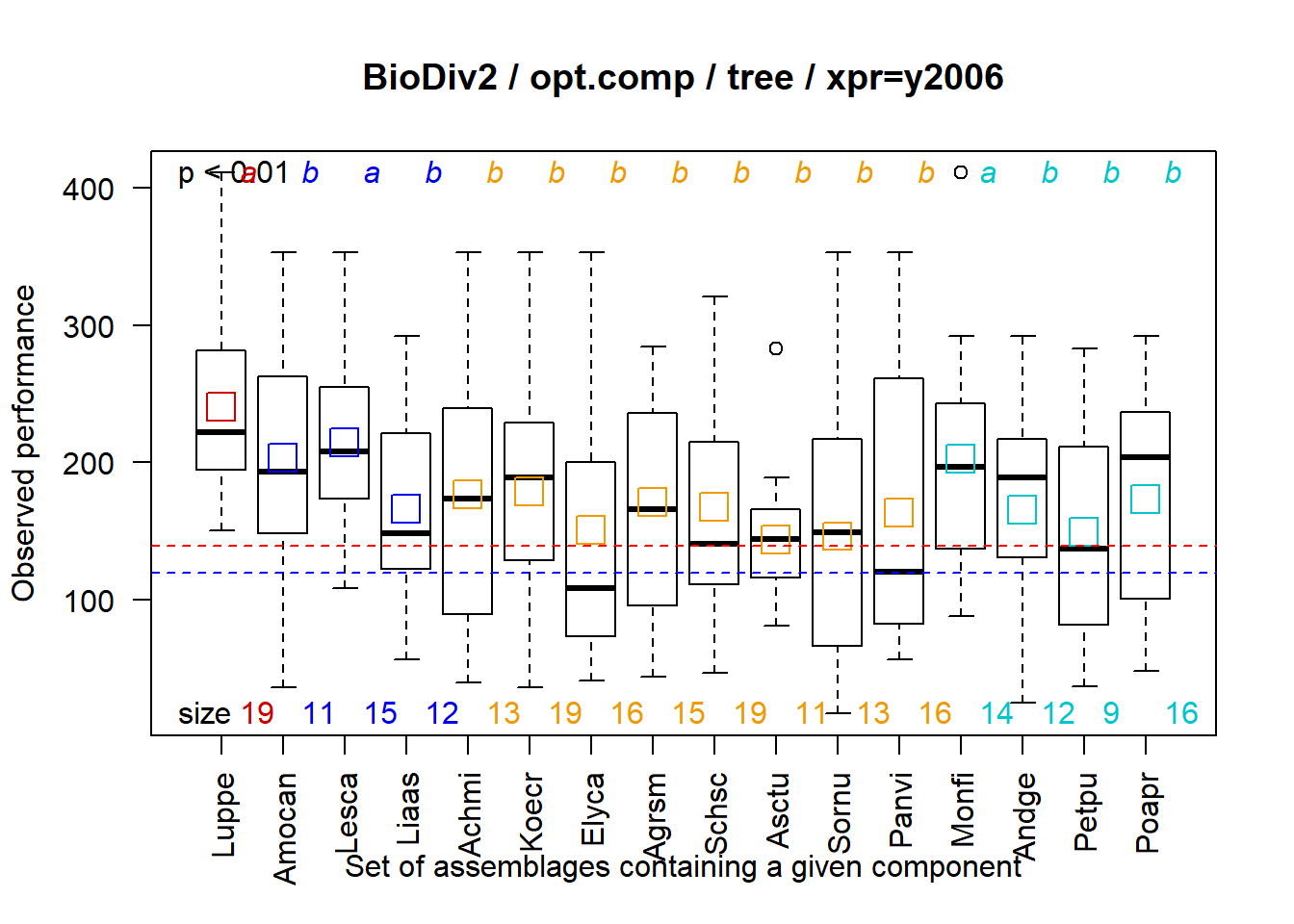Opt.comp = list(‘tree’) plots performances over time sorted by component clusters as in hierarchical tree